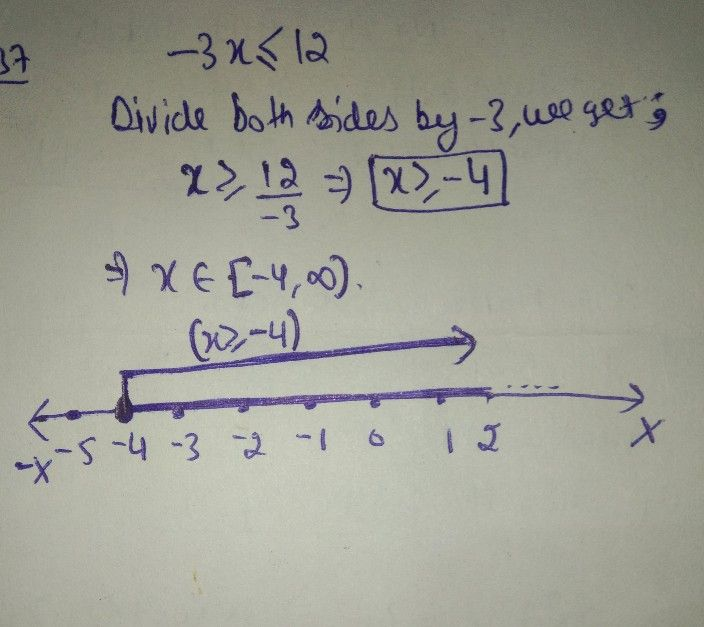Symbol
ProblemQuestion $1$ $\left(a\right)$ Solve the following $AN$ AND graph the solution on a number line. $\left(3marks\right)$ $-3x\leq 12$
Other
SolutionQanda teacher - Akshay
HiAll the best